Find solution of equations- Equations given

Chapter 4 Class 12 Determinants
Concept wise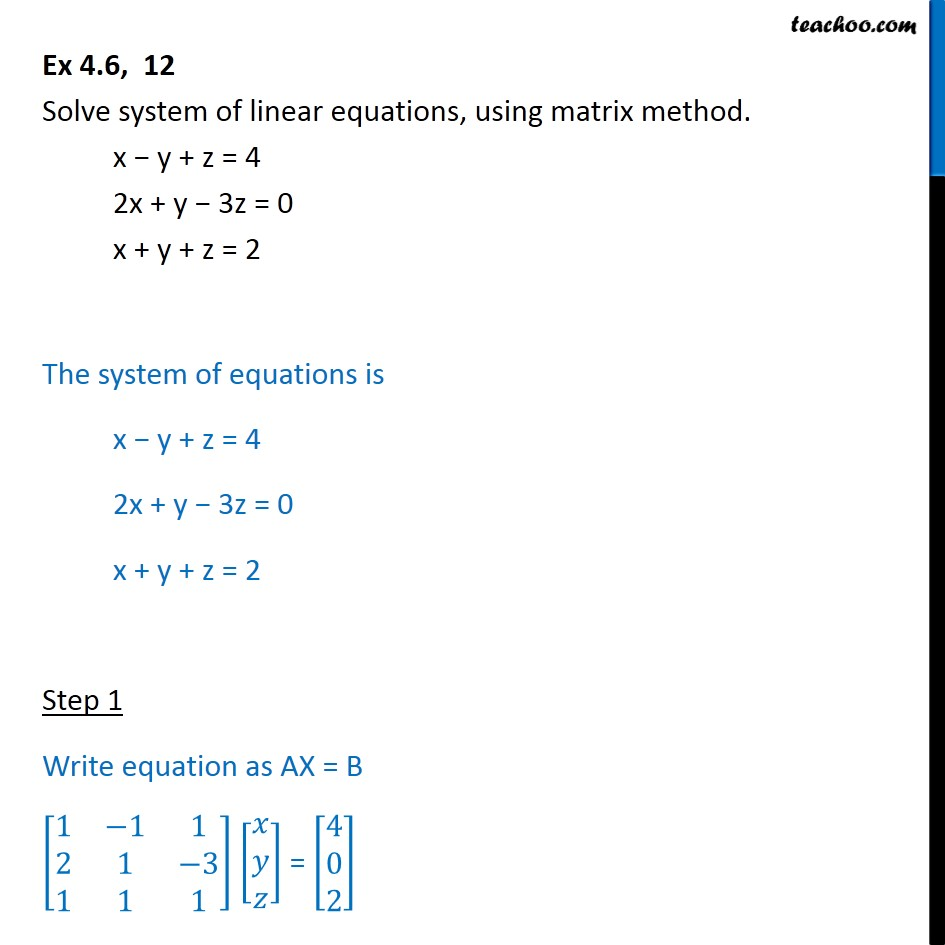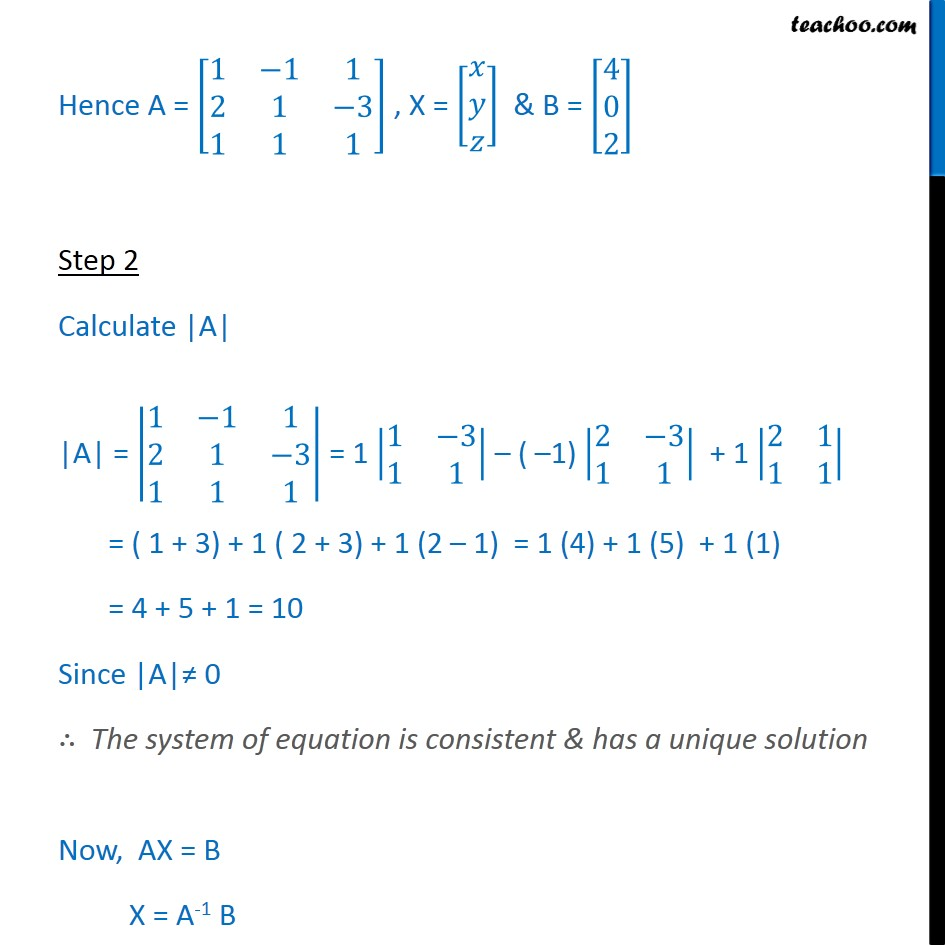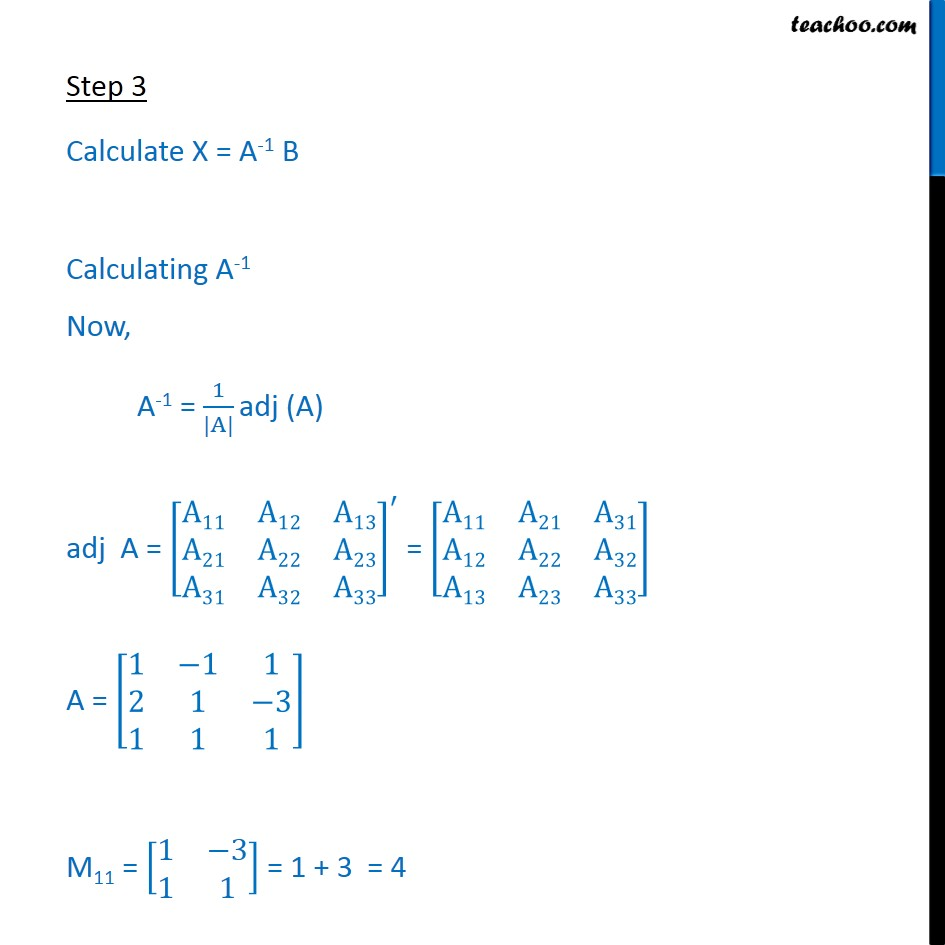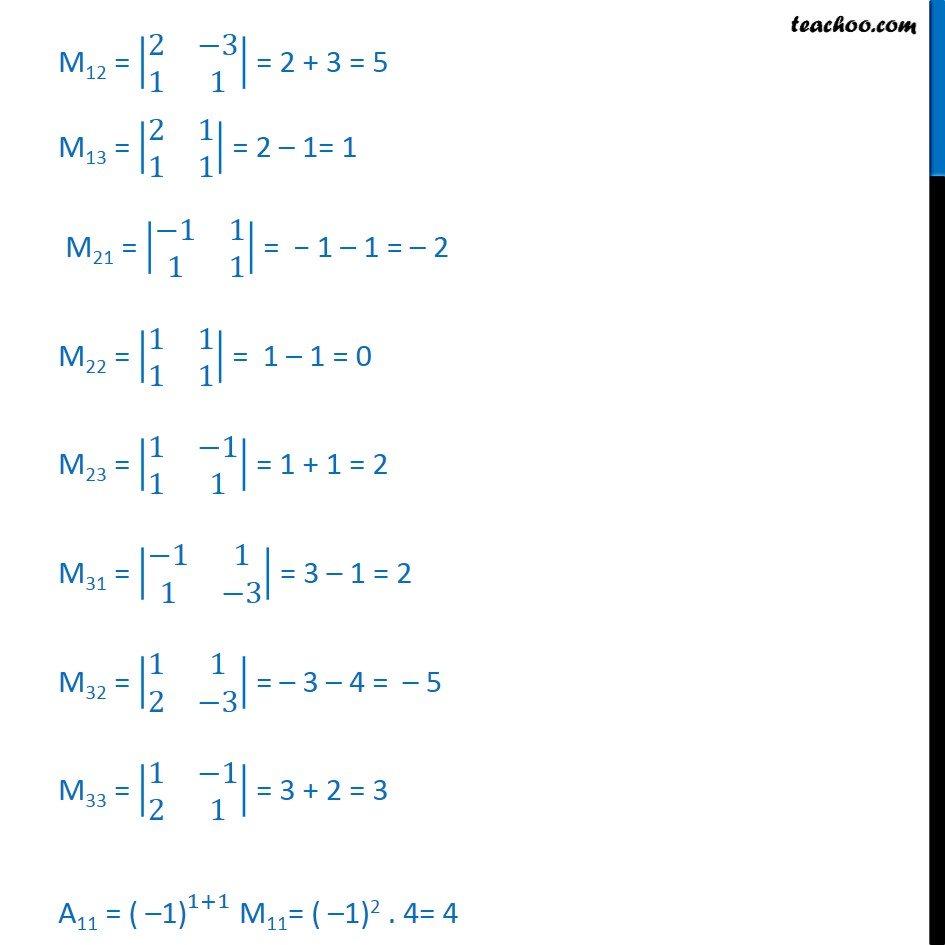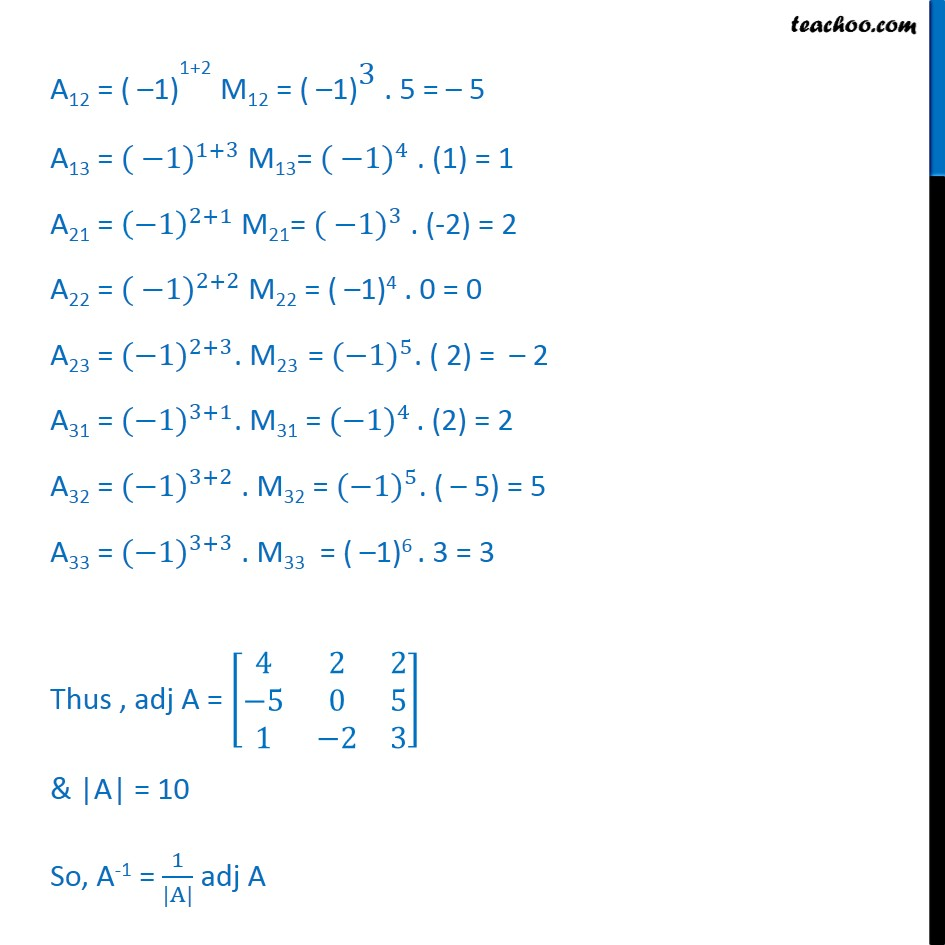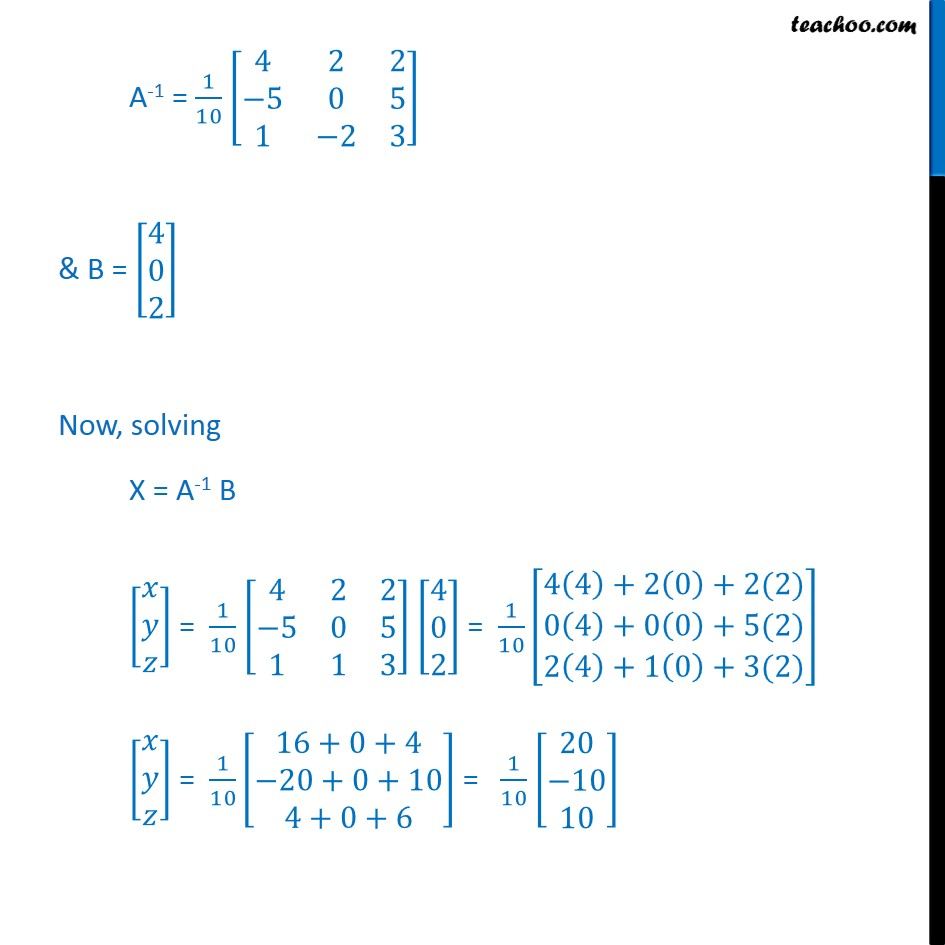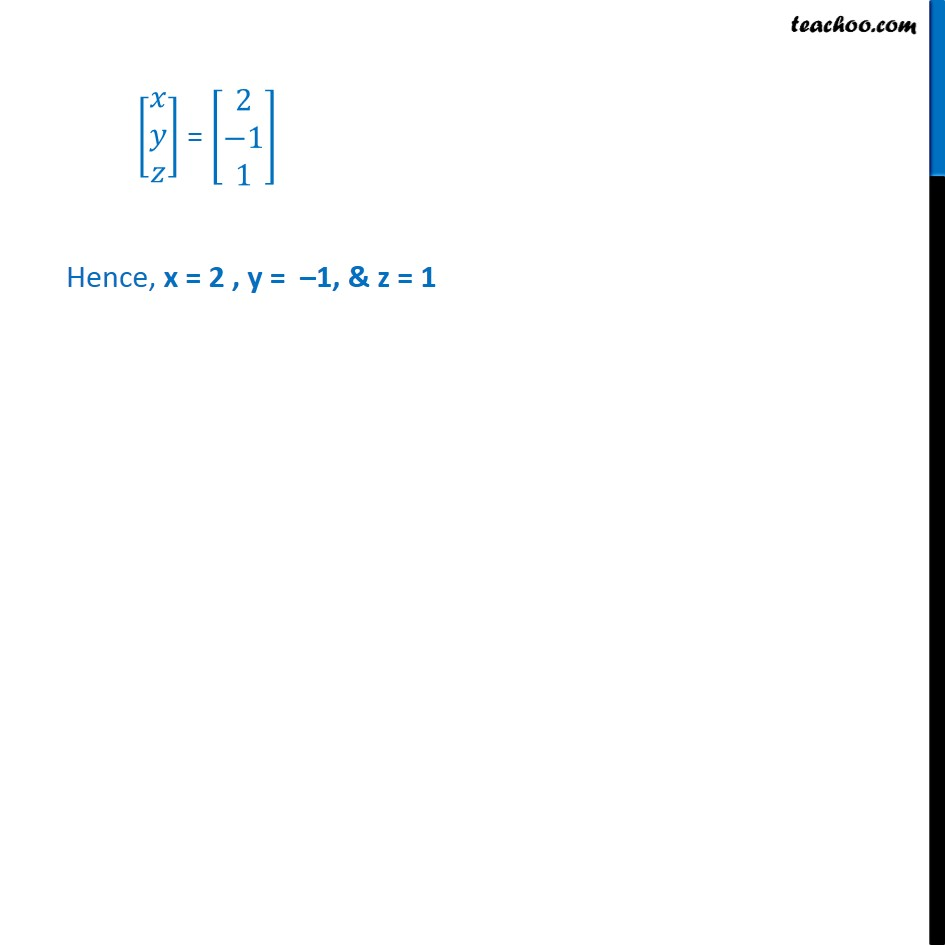Learn in your speed, with individual attention - Teachoo Maths 1-on-1 Class

### Transcript

Ex 4.5, 12 Solve system of linear equations, using matrix method. x y + z = 4 2x + y 3z = 0 x + y + z = 2 The system of equations is x y + z = 4 2x + y 3z = 0 x + y + z = 2 Step 1 Write equation as AX = B 1 1 1 2 1 3 1 1 1 = 4 0 2 Hence A = 1 1 1 2 1 3 1 1 1 , X = & B = 4 0 2 Step 2 Calculate |A| |A| = 1 1 1 2 1 3 1 1 1 = 1 1 3 1 1 ( 1) 2 3 1 1 + 1 2 1 1 1 = ( 1 + 3) + 1 ( 2 + 3) + 1 (2 1) = 1 (4) + 1 (5) + 1 (1) = 4 + 5 + 1 = 10 Since |A| 0 The system of equation is consistent & has a unique solution Now, AX = B X = A-1 B Step 3 Calculate X = A-1 B Calculating A-1 Now, A-1 = 1 |A| adj (A) adj A = A11 A12 A13 A21 A22 A23 A31 A32 A33 = A11 A21 A31 A12 A22 A32 A13 A23 A33 A = 1 1 1 2 1 3 1 1 1 M11 = 1 3 1 1 = 1 + 3 = 4 M12 = 2 3 1 1 = 2 + 3 = 5 M13 = 2 1 1 1 = 2 1= 1 M21 = 1 1 1 1 = 1 1 = 2 M22 = 1 1 1 1 = 1 1 = 0 M23 = 1 1 1 1 = 1 + 1 = 2 M31 = 1 1 1 3 = 3 1 = 2 M32 = 1 1 2 3 = 3 4 = 5 M33 = 1 1 2 1 = 3 + 2 = 3 A11 = ( 1) 1+1 M11= ( 1)2 . 4= 4 A12 = ( 1) 1+2 M12 = ( 1) 3 . 5 = 5 A13 = ( 1) 1+3 M13= ( 1) 4 . (1) = 1 A21 = 1 2+1 M21= ( 1) 3 . (-2) = 2 A22 = ( 1) 2+2 M22 = ( 1)4 . 0 = 0 A23 = ( 1) 2+3 . M23 = ( 1) 5 . ( 2) = 2 A31 = ( 1) 3+1 . M31 = ( 1) 4 . (2) = 2 A32 = ( 1) 3+2 . M32 = ( 1) 5 . ( 5) = 5 A33 = ( 1) 3+3 . M33 = ( 1)6 . 3 = 3 Thus , adj A = 4 2 2 5 0 5 1 2 3 & |A| = 10 So, A-1 = 1 |A| adj A A-1 = 1 10 4 2 2 5 0 5 1 2 3 & B = 4 0 2 Now, solving X = A-1 B = 1 10 4 2 2 5 0 5 1 1 3 4 0 2 = 1 10 4 4 +2 0 +2(2) 0 4 +0 0 +5(2) 2 4 +1 0 +3(2) = 1 10 16+0+4 20+0+10 4+0+6 = 1 10 20 10 10 = 2 1 1 Hence, x = 2 , y = 1, & z = 1